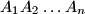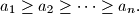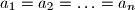IMO Shortlist 1963 problem 3

Kvaliteta:
Avg: 0,0
Težina:
Avg: 0,0
In an$n$-gon$A_{1}A_{2}\ldots A_{n}$, all of whose interior angles are equal, the lengths of consecutive sides satisfy the relationProve that$a_{1}=a_{2}= \ldots= a_{n}$.
Izvor: Međunarodna matematička olimpijada, shortlist 1963